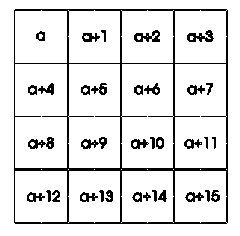#### You may also like### Lastly - Well

What are the last two digits of 2^(2^2003)?### How Many Miles to Go?

How many more miles must the car travel before the numbers on the milometer and the trip meter contain the same digits in the same order?### Pyramids

What are the missing numbers in the pyramids?

# Always the Same

##### Age 11 to 14 Challenge Level:

Thank you to Jimmy, Natalie and Lucy at West Flegg GM Middle School for their work on the problem "Always the Same". Natalie is not the first student to add together all the numbers 1 through 16 and take the average.

Thus replacing the ascending numbers with 8.5 in every cell and circling four cells gives a total of 34. Or as Natalie did, she realised that "you pick numbers from each column and row" and took the average between the sum of the four columns:

i.e. (28 + 32 + 36 + 40)/4 = 34

A good solution with this method came from Melanie and Rachel of Flegg High School.

A proof of this problem could be as follows.

Let the first number be a.Then when choosing numbers from rows and column that do not coincide we have: a + (a + 4) + (a + 8) + (a + 12) + 1 + 2 + 3 = 'Magic number' We add 1 because there is one number in the second column, 2 because there is one number in the third column and 3 because there is one number in the fourth column.

Hence:

4a + (4 + 8 + 12) + ( 1 + 2 + 3) = 34
i.e. 4a + 30 = 34
i.e a = 1
and the array is 1 through 16 as set.

But suppose the 'magic number' had been 62 then

4a + 30 = 62
i.e a = 8
and the array would have been 8 through 23.

Hope that the explanation above helps especially Josh at Russell Lower School to "work out where we went wrong".

We could have used a 5 by 5 array of ascending numbers!